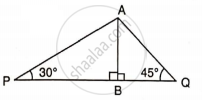# Find Pq, If Ab = 150 M, ∠P = 30o and ∠Q = 45°. - Mathematics

Sum

Find PQ, if AB = 150 m, ∠ P = 30° and ∠ Q = 45°.#### Solution

From ΔAPB

tan 30° = "AB"/"PB"

(1)/(sqrt(3)) = (150)/"PB"

PB = 150sqrt(3)

PB = 259.80 m

Also, from ΔABQ

tan 45° = "AB"/"BQ"

1 = (150)/"BQ"

BQ = 150 m

Therefore,

PQ = PB + BQ

PQ = 259.80 + 150

PQ = 409.80 m

Concept: Solution of Right Triangles
Is there an error in this question or solution?

#### APPEARS IN

Selina Concise Mathematics Class 9 ICSE
Chapter 24 Solution of Right Triangles [Simple 2-D Problems Involving One Right-angled Triangle]
Exercise 24 | Q 18.1 | Page 304